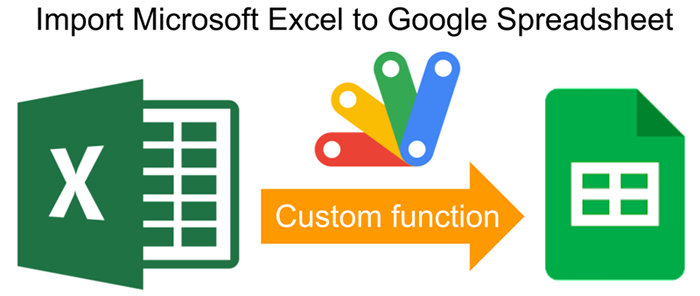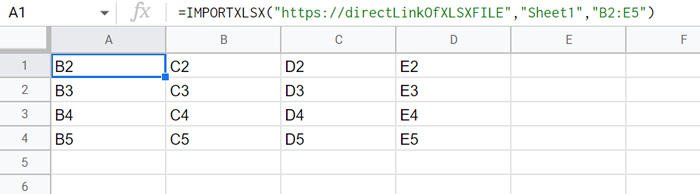GistsThis is a sample script for importing Microsoft Excel (XLSX) data to Google Spreadsheet using a custom function with Google Apps Script.

## Usage

### 1. Install SheetJS library.

Please copy the script of the SheetJS library from https://cdn.sheetjs.com/xlsx-latest/package/dist/xlsx.full.min.js, and paste the script to the script editor of Google Spreadsheet, and save the script.

In this case, I would like to recommend the following flow.

1. Add a new script to the script editor. For example, the filename is SheetJS.
2. Copy and paste the script of https://cdn.sheetjs.com/xlsx-latest/package/dist/xlsx.full.min.js to the added script file, and save the script.
3. Copy and paste the following sample script of the custom function to the other script file (It’s the default script file (Code.gs)).

### 2. Prepare custom function.

/**
* Retrieve values from XLSX data of the direct link of the XLSX file.
* @param {string} url Direct link of XLSX file.
* @param {string} sheetName Sheet name.
* @param {string} range Range as A1Notation except for sheet name.
* @return {Array} Retrieved values.
* @customfunction
*/
function IMPORTXLSX(url, sheetName, range) {
const res = UrlFetchApp.fetch(url, { muteHttpExceptions: true });
if (res.getResponseCode() != 200) {
throw new Error(
);
}
const book = XLSX.read(new Uint8Array(res.getContent()), { type: "array" });
const worksheet = book.Sheets[sheetName];
if (!range) {
const csv = XLSX.utils.sheet_to_csv(worksheet);
const values = Utilities.parseCsv(csv);
return values;
}
var rng = XLSX.utils.decode_range(range);
const values = [];
for (let row = rng.s.r; row <= rng.e.r; row++) {
const temp = [];
for (let col = rng.s.c; col <= rng.e.c; col++) {
const v = XLSX.utils.encode_cell({ r: row, c: col });
temp.push(worksheet[v] ? worksheet[v].v : null);
}
values.push(temp);
}
return values;
}


### 3. Testing.When you use this script, please put the following custom function into a cell. In this script, the arguments of 1st to 3rd are the direct link of the XLSX file, the sheet name, and the range of A1Notation except for the sheet name, respectively. When the range is not used, all values of the sheet are returned.

=IMPORTXLSX("###Direct link of XLSX file###", "Sheet1")

=IMPORTXLSX("###Direct link of XLSX file###","Sheet1","B2:E5")


## Note

• In this script, if you don’t want to copy and paste the SheetJS library to the script editor, you can also install it with the following script. But, in this case, the process cost becomes high. Please be careful about this.

const cdnjs =
"https://cdn.sheetjs.com/xlsx-latest/package/dist/xlsx.full.min.js";
eval(UrlFetchApp.fetch(cdnjs).getContentText());